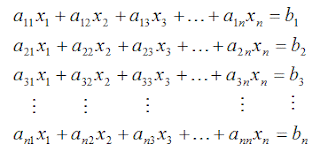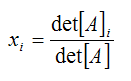Improve math skills of your kids - Learn step-by-step arithmetic from Math games

Math: Unknown - Step-by-step math calculation game for iOS.

Math: Unknown is much more than a math game. It is a step-by-step math calculation game which will teach users how to calculate in the correct order rather than just asking only the final calculated results.

The app consists of four basic arithmetic operations which are addition, subtraction, multiplication and division. In order to get started, users who are new to arithmetic can learn from animated calculation guides showing step-by-step procedures of solving each type of operation. It is also helpful for experienced users as a quick reference.

Generally, addition and subtraction may be difficult for users who just start learning math especially when questions require carrying or borrowing (also called regrouping). The app helps users to visualize the process of carrying and borrowing in the way it will be done on paper. Once users understand how these operations work, they are ready to learn multiplication and division.

For most students, division is considered as the most difficult arithmetic operation to solve. It is a common area of struggle since it requires prior knowledge of both multiplication and subtraction. To help users understand division, the app uses long division to teach all calculation procedures. Relevant multiplication table will be shown beside the question. Users will have to pick a number from the table which go into the dividend. Multiplication of selected number and divisor is automatically calculated, but the users have to do subtraction and drop down the next digit themselves. Learning whole calculation processes will make them master it in no time.

Math: Unknown is a helpful app for students who seriously want to improve arithmetic calculation skills.

Solving System of Equations using Gauss Elimination Method (Part 1)Sometimes engineers need to solve a system of equations to obtain solutions for their engineering design works. This kind of problems consists of several number of unknowns and equations that cannot be solved easily without good knowledge of system of equations solving method and understanding of computer programming. If we need to solve a set of linear equations with 2 - 3 unknowns, it will be easily to solve by hand calculation. There are several methods available for solving them. But when we need to solve much more unknowns e.g. 20 unknowns or 1,000 unknowns, computer software is unavoidable.

System of equations with "n" linear equations can be expressed as follows:We can write it the matrix form as [A]{X} = {B}

The dimension of matrix [A] is (nxn), vector {X} is (nx1) and vector {B} is (nx1).
There are several methods to solve a set of simultaneous equations e.g. Cramer's rule, Gauss elimination or Gaussian Elimination method, Gauss-Jordon method, matrix inversion method, LU decomposition method, Cholesky decomposition method, etc.

Cramer's rule can easily solve a set of equations with small number of equation. The Cramer's rule uses determinant to solve for the unknowns. The unknowns can be solved from:However, the number of calculations using Cramer's rule is approx. (n-1)(n+1)!, where n is number of equations to be solved. Therefore, if we want to solve a set of 10 linear equations, the number of calculations will be approx 360,000,000 times. But if we use Gauss Elimination method for 100 linear equations, the number of calculations is only about 700,000. Thus, practically, Gauss Elimination Method is the most favorite method for general use.

Gauss Elimination Method or Gaussian Elimination Method is widely used for solving most of engineering problems. It can be used to program in the computer easily. Gauss Elimination method can be categorized as follows:
1. Forward elimination
2. Back substitution
If you're interested in more details, please check the link of article sources below.

Mechanical Design Handbook has developed a VBA excel program to solve a set of simultaneous equations using original FORTRAN code from "Numerical Methods in Engineering by Dr. Pramote Dechaumphai". The program is written in VBA excel with simple user interface. It can solve up to 10 numbers of equations. Mechanical Design Handbook is going to share more details about how to use the program and download link in later posts [Solving System of Equations using Gauss Elimination Method (Part 2)].

Here are some pictures of the program that we will discuss in more details later.

Main screen

User interface

Input and Solution Screen

Source: